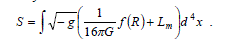ISSN ONLINE(2319-8753)PRINT(2347-6710)

All submissions of the EM system will be redirected to Online Manuscript Submission System. Authors are requested to submit articles directly to Online Manuscript Submission System of respective journal.

# Bianchi Type-I (Kasner Form) Cosmological Model in F(R) Theory of Gravity

 L. S. Ladke Associate Professor & Head, Department of Mathematics, Sarvodaya Mahavidyalaya, Sindewahi, Dist:Chandrapur (441222), India. Related article at Pubmed, Scholar Google

Visit for more related articles at International Journal of Innovative Research in Science, Engineering and Technology

## Abstract

The vacuum solution of Bianchi types-I (Kasner form) cosmological model in f(R) theory of gravity has been obtained. The general solution of the field equations in respect of Bianchi type-I space-time in Kasner form have been obtained with the assumption of special form of deceleration parameter. The physical behavior of the model has been discussed by obtaining some physical quantities.

### Keywords

f (R) theory of gravity, Bianchi type-I space-time in Kasner form, Special form of deceleration parameter.

### INTRODUCTION

The f (R) theory of gravity plays an important role in describing the evolution of the universe. The f (R) theory of gravity is the modification of the general theory of relativity proposed by Einstein. The f (R) actions were first studied by Weyl  and Eddington . The f (R) gravity provides a very natural unification of the early-time inflation and late-time acceleration. Carroll et al. explained the presence of a late time cosmic acceleration of the universe in f (R) gravity. As per Nojiri and Odintsov ( , ) a unification of the early time inflation and late time acceleration is allowed in f (R) gravity.

### RELATED WORK

Multamaki & Vilja ( , ) investigated static spherically symmetric vacuum solutions of the field equations and non-vacuum solutions by taking perfect fluid respectively. Sharif & Shamir  studied exact vacuum solutions of Bianchi type-I & type-V space times in f (R) theory of gravity. Non-vacuum solutions in Bianchi type-I & type-V using perfect fluid in f (R) gravity have been obtained by Sharif & Shamir . Shamir  discussed the plane symmetric vacuum Bianchi type-III cosmology in f (R) gravity. Bianchi type-III space time with anisotropic fluid in f (R) gravity has been dealt with by Sharif & Kausar . Aktas et al.  have studied anisotropic models in f (R) theory of gravity. Adhav  discussed the Kantowski–Sachs string cosmological model in f (R) theory of gravity. Singh et al.  studied functional form of f (R) with power-law expansion in anisotropic model. Recently, Reddy et al.  studied vacuum solution of Bianchi type-I and V models in f (R) gravity with a special form of deceleration parameter.
Motivated by the above research, in this paper, the general solutions of the field equations of f (R) gravity for Bianchi type-I space-time in Kasner form have been obtained with the assumption of special form of deceleration parameter. The physical behavior of the model is also discussed.

### f (R) THEORY OF GRAVITY AND DECELERATION PARAMETER

The f (R) theory of gravity is nothing but modification of general theory of relativity. The action for f (R) theory of gravity is given byiv) It is observed that in Bianchi type-I model, the anisotropy increases as time increases and then it decreases to zero after some time and remains zero. Hence, the model reaches to isotropy after some finite time.
v) It is seen that, the results obtained here are similar to the results obtained earlier by Reddy et al...

### References

 Weyl H.: Ann. Phys., 59, 101 (1919).

 Eddington A.S.: The mathematical theory of relativity (Cambridge university press, Cambridge, (1923)

 Carroll, S.M., et al. “Is cosmic speed-up due to new gravitational physics?” Phys. Rev. D, 70, pp.043528, (2004).

 Nojiri S and Odintsov S D “Modified gravity and its reconstruction from the universe expansion history” J. Phys. Conf.Ser. 66, 012005,(2007).

 Nojiri S and Odintsov S D . “ Future evolution and finite-time singularities in F(R) gravity unifying inflation and cosmic acceleration” Phys. Rev. D, 78, 046006, (2008).

 Multamaki,T., Vilja, I. “Spherically symmetric solutions of modified field equations in f(R) theories of gravity” Phys. Rev. D 74, 064022 (2006).

 Multamaki,T., Vilja, I. “Static spherically symmetric perfect fluid solutions in f(R) theories of gravity” Phys. Rev. D 76, 064021(2007).

 Sharif,M.,Shamir,M.F. : Class. Quantum Grav. 26 , 235020 (2009).

 Sharif,M.,Shamir,M.F. “Energy distribution in f (R) gravity” Gen. Relativ. Grav. 42, pp.1557-1569 (2010).

 Shamir,M.F. “Plane Symmetric Vacuum Bianchi Type III Cosmology in f(R) Gravity” Int. J. Theor. Phys. 50, pp.637-643 (2011).

 Sharif, M., Kausar, H.R.” Anisotropic fluid and Bianchi type III model in f (R) gravity” Phys. Lett. B, 697, pp.1 6, (2011).

 Aktas, C., et al.” Behaviors of dark energy and mesonic scalar field for anisotropic universe in f (R) gravity” Phys. Lett. B,707, pp.237-242 (2012).

 Adhav, K.S.” Kantowski–Sachs string cosmological model in f(R) theory of gravity” Can.J.Phys.,90,pp.119-123, (2012).

 Singh,V . and Singh, C.P. “Functional form of f(R) with power-law expansion in anisotropic model” Astrophys Space Sci, 346, pp.285- 289, (2013).

 Reddy, DRK et al.” Vacuum Solutions of Bianchi Type-I and V Models in f(R) Gravity with a Special Form of Deceleration Parameter” Int.Jou.Sci.Adv.Tech., 4, 3, pp.23-33 (2014).

 Berman, M.S.” A special law of variation for Hubble’s parameter” Nuovo Cimento B, 74, 2, pp.182-186, (1983)

 Akarsu O.and Kilinc C.B. “Bianchi type III models with anisotropic dark energy” Gen. Relat. Gravit. 42, pp.763-775 (2010).

 Pradhan et al.” Bianchi Type-I Anisotropic Dark Energy Model with Constant Deceleration Parameter” Int J Theor Phys, 50(9), pp.2923-2938 (2011).

 Adhav. K.S. et al. “Bianchi type-VI0 cosmological models with anisotropic dark energy” Astrophysics and Space Science, 332(2), pp.497-502(2011).

 Adhav. K.S. et al. “Kantowski-Sachs cosmological models with anisotropic dark energy” Cent. Eur. J. Phys., 9(4) , pp.919-925 (2011).

Cunha J.V. and Lima J.A.S.” Transition redshift: new kinematic constraints from supernovae” Mon. Not. R. Astron. Soc.: 390(1),pp.210-217 (2008).

 Singha A.K. and Debnath U.“Accelerating Universe with a Special Form of Decelerating Parameter” Int. J. Theor Phys., 48(2), pp.351-356 (2009).

 Kotub Uddin et al. “Cosmological perturbations in Palatini-modified gravity” Class. Quantum Grav. 24 , pp.3951-3962 (2007).